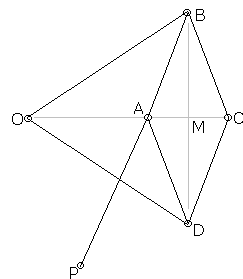Peaucellier, Captain Charles-Nicolas,
French engineer who in 1873 invented a linkage, known as the Peaucellier straight-line mechanism, capable of describing a circle of any radius, including an infinite one - straight-line.
From Encyclopaedia Britannica

Yom Tov Lipman Lipkin (1846-1876),
Lithuanian Jewish mathematician and inventor,
wikipedia, Jewish Encyclopedia

The Java simulation below allows one to convert circular motion into linear. The Peaucellier device consists of 7 rods linked at their ends. Four form a diamond, two more (of equal length) joint at one end are attached to two opposite vertices of the diamond. One rod joins the center of a circle (actually the circle is supposed to be invisible) to a third vertex of the diamond. When this third vertex slides along the circle, the remaining vertex traces a straight line.

The simulation has several control points each indicated by a small ring. Except for the third vertex, dragging these changes configuration of the device. Drag the third vertex over the circle and the free vertex will draw a straight line.

Well, it's supposed to be straight and I can prove that it should be straight. But what can one expect of analog devices. Inevitable rounding errors crop up creating an effect of randomness. Sometimes it's more pronounced - and you are invited to think of when this happens. When the drawing vertex goes to infinity the rounding errors result in spurious straight lines. I could have precluded this from happening but decided against it. Once warned, you know what to expect, whereas I have enjoyed unfettered motion of the rhombus.

This applet requires Sun's Java VM 2 which your browser may perceive as a popup. Which it is not. If you want to see the applet work, visit Sun's website at https://www.java.com/en/download/index.jsp, download and install Java VM and enjoy the applet.

 What if applet does not run?

The theory of the device depends on the notion of (circle) inversion - reflection in a circle.Seven rods are linked by moving joints at points O, P, A, B, C, and D. (The gray lines are not part of the linkage.) Rods AB, BC, CD, and AD are equal, as are the rods OB and OD. The figure ABCD is always a rhombus, while the rods OB and OD keep the vertices A and C aligned with O. Let M be the mid point of AC. Then

 OA·OC = (OM - MA)·(OM + MC) = (OM - MA)·(OM + MA) = OM2 - MA2 = OM2 + MB2 - MB2 - MA2 = OB2 - AB2,

which is constant as long as the lengths of rods OB and AB are fixed. This means that the points A and C are always inverses of each other with center O. In other words, the points A and C trace two curves that are inverse images of each other. If now AP = OP, then A traces a circle that passes through the center of inversion. Such circles are mapped onto straight lines, the one of which is traced by the point C. (In reality, C only traces a line segment.)

Playing with this device provides an endless stream of questions and problems in an Analytic Geometry class. Just think of how the moving points relate to each other.

Please note that inaccuracies in the drawing of the straight line demonstrate just one more time that Geometry can't depend on analog devices. Geometry is a deductive science whose objects are absolutely abstract. Pictures and figures in the manuals serve only to illustrate, never to prove a proposition. In geometric applications, the more accurate approximation to an abstraction, the better it confirms with the theoretical argument.

As an historic diversion, the question of converting circular motion into rectilinear was raised in the first half of the 19th century. The original stimulus was a study of the modes of action of James Watt's steam engine [Grattan-Guinness, p.596]. No less a mathematician than P. Chebyshev thought that this is impossible. In the 1850s he sought a linkage that would provide an approximate solution. This led to his discovery of the polynomials now named after him. The polynomials have the property of providing the least uniform deviation from the straight line among all polynomials of the given degree with the leading coefficient equal to 1. A 7 rods solution was found by his student L. Lipkin in the early 1870s, about a decade after the discovery of Peaucellier.

(An even simpler device is known as Hart's inversor.)

References

1. I. Grattan-Guinness, The Rainbow of Mathematics, W. W. Norton & Co, 2000Inversion - Introduction